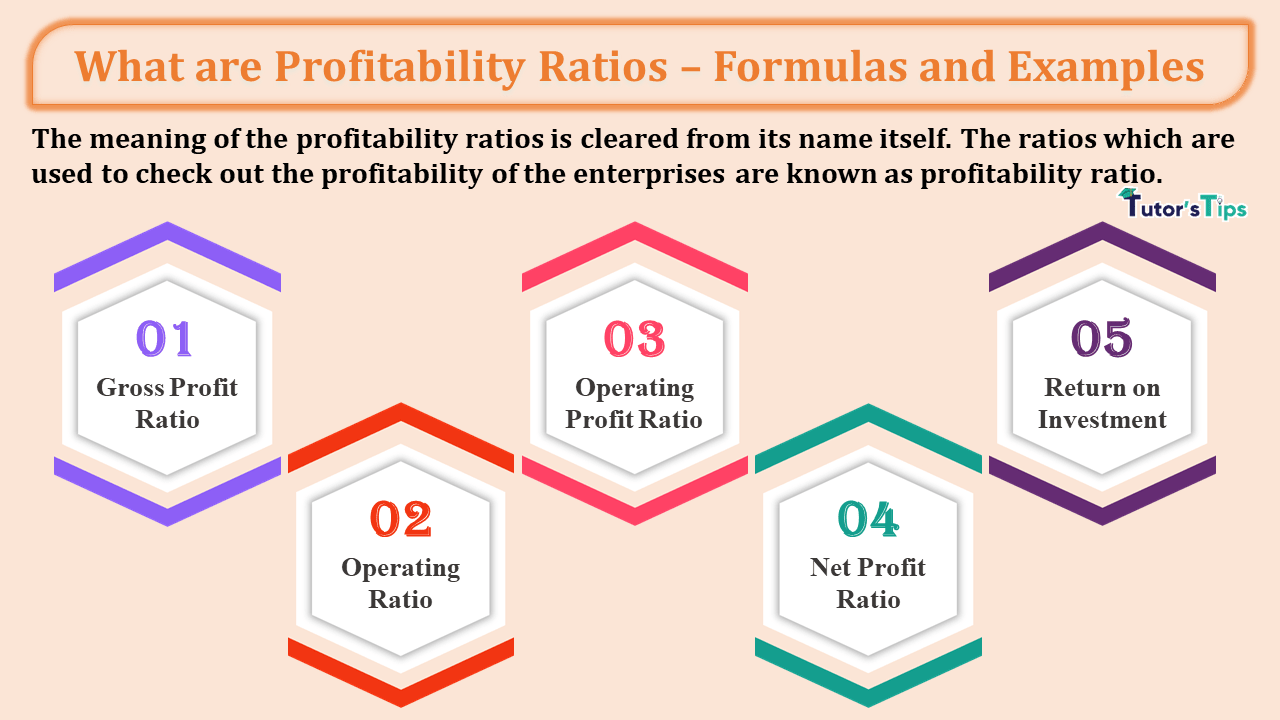# What are Profitability Ratios – Formulas and ExamplesWhat are Profitability Ratios – Formulas and Examples-min

Profitability Ratios are used to know the profitability of the business. It covers five types of ratios which are discussed in this article.

## Meaning of Profitability Ratios:

The meaning of the profitability ratios is cleared from its name itself. The ratios which are used to check out the profitability of the enterprises are known as profitability ratio. It gets to know to related parties the actual Profitability of the business.

## Why do we need to Calculate Profitability Ratios?

Profitability Ratios are needed to check out the actual profitability of the business. These all ratios calculated in the form of a percentage. These all are benefited in the same way(except operating Ratio). It means if we got a high percentage then these are better for business enterprise and vice versa. Operating Ratio is low in percentage is better for business and vice versa.

## The formula of Profitability Ratios:

To Check out the Profitability of the business we can use the following five formulas:

### 1. Gross Profit Ratio:

The Gross Profit Ratio is used to know the relationship between the Revenue from Operation and Gross Profit during the year of the business.

###### Formula to Calculate Gross Profit Ratio:
 Gross Profit Ratio = Gross Profit X 100 Revenue from Operation

##### We will get a ratio in percentage.

Now the question is how to calculate the Gross Profit:

= Total Revenue from an operation – Cost of Revenue from Operation

Now the question is how to calculate the Cost of Revenue from Operation:

= Opening Inventory + Net Purchase + Direct Expenses – Closing Inventory

OR

Total Revenue from an operation – Gross Profit

Note: – Total Revenue from operation means Net Sales of the business.

### 2. Operating Ratio:

The Operating Ratio is used to know the relationship between the Revenue from Operation and Operating cost during the year of the business.

###### Formula to Calculate Operating Ratio:
 Operating Ratio = Operating Cost X 100 Revenue from Operation
##### We will get a ratio in percentage.

Now the question is how to calculate the Operating Cost:

= Opening Inventory + Net Purchase + Direct Expenses + operating expenses – Closing Inventory

OR

Total Revenue from an operation – Gross Profit

Note: – Operating expenses include the following expenses:

• Employees Benefit Expenses
• Depreciation and Amortisation expenses
• Other Expenses (Other than Non-operating Expenses)

### 3. Operating Profit Ratio:

The Operating Profit Ratio is used to know the relationship between the Revenue from Operation and Operating Profit during the year of the business.

###### Formula to Calculate Operating Profit Ratio:
 Operating Profit Ratio = Operating Profit X 100 Revenue from Operation
##### We will get a ratio in percentage.

Now the question is how to calculate the Operating Profit:

= Gross Profit + Other Operating Income – Other Operating Expenses

Or

= Net Profit (Before Tax) + Non-Operating Expenses/Losses – Non-Operating Incomes

Or

= Revenue From Operation – Operating Cost

Note: – Total Revenue from operation means Net Sales of the business.

### 4. Net Profit Ratio:

The Net Profit Ratio is used to know the relationship between the Revenue from Operation and Net Profit during the year of the business.

###### Formula to Calculate Net Profit Ratio:
 Net Profit Ratio = Net Profit X 100 Revenue from Operation
##### We will get a ratio in percentage.

Now the question is how to calculate the Net Profit:

= Revenue from Operations – Cost of Revenue from Operations – Operating Expenses – Non-Operating Expenses + Non-operating Income – Tax

Or

= Revenue From Operation – Total Cost of goods sold

Note: – Total Revenue from operation means Net Sales of the business.

### 5. Return on Investment (ROI):

The Return on Investment is used to know the relationship between the Capital Employed and Net Profit before interest, tax and dividend during the year of the business.

###### Formula to Calculate Return on Investment (ROI):
 Return on Investment = Net Profit before interest, tax and dividend X 100 Capital Employed
##### We will get a ratio in percentage.

Now the question is how to calculate the Net Profit:

= Revenue from Operations – Cost of Revenue from Operations – Operating Expenses – Non-Operating Expenses(excluding interest) + Non-operating Income

Or

= Revenue From Operation – Total Cost of goods sold

Now the question is how to calculate the Capital Employed:

If the liabilities side approach is followed:

= Shareholder Funds + Non-Current Liabilities

If the assets side approach is followed:

= Non-Current Assets + Working Capital